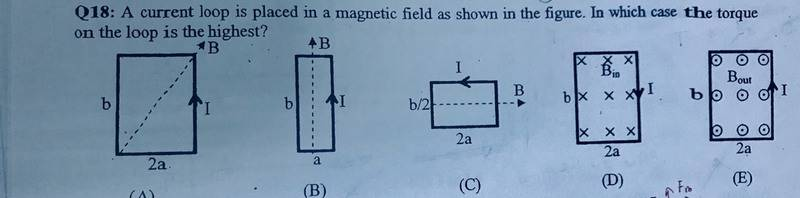# The case that has the highest torque on the loop

## Homework Statement## Homework Equations

T = IAB sin θ ; θ is the angle between B and I.
T = μ × B

## The Attempt at a Solution

A) T = IAB sin θ
T = √2 a b I B (θ = 45°)
B) T = 0 , because θ = 0
C) T = 0 , because θ =180°
D) T = μ × B
The direction of μ = -k , because the fingers points to the direction of current and the thump points to the direction of T.
T = (-k) × (-k)
=0
E) T = (k)×(k)
=0
So , the answer is 'A' .
Is it correct ?

#### Attachments

Unfortunately, no. :)

In your analysis of A, B, and C, you're only accounting for one side of each rectangle--the one labeled with an "I" in the diagram. In fact, in the formula ##| \mathbf{\tau} | = IAB \sin(\theta)##, the angle ##\theta## is meant to be taken between the current loop's normal vector and the magnetic field, *not* between the current and ##\mathbf{B}##. This is actually a consequence of the second formula you gave, ##\mathbf{\tau} = \mathbf{\mu} \times \mathbf{B}##, since the magnetic moment of a current loop is ##IA \mathbf{n}## (where ##\mathbf{n}## is the positively-oriented unit norm to the loop).

So actually, ##\theta = 90^{\circ}## in each of choices A, B, and C. Given this, what should the answer be?

Last edited:
So actually, ##\theta = 90^{\circ}## in each of choices A, B, and C.
And in 'D' and 'E' , ##\theta = 0^{\circ}## ?

In your analysis of A, B, and C, you're only accounting for one side of each rectangle--the one labeled with an "I" in the diagram.
Should I multiply by 4 ?

In D and E, ##\theta## is either ##0## or ##180^{\circ}##; in either case the torque vanishes.

Multiplying by 4 won't solve the problem. The issue is that the magnetic field makes a different angle with each of the sides of the loop. The correct way to account for this is by using the magnetic moment of the loop *as a whole* instead of trying to add up the torques on each side.

The correct way to account for this is by using the magnetic moment of the loop *as a whole*
T = μ × B
= I A B sin θ
The area is 2a*b and the angle is 90°.
So, T = 2 I a b B
Right ?